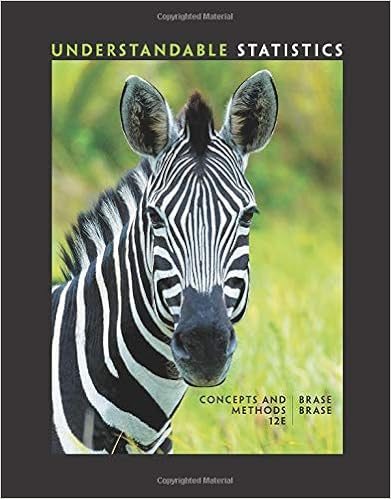# B find the probability that a randomly selected adult

• Test Prep
• 7
• 90% (21) 19 out of 21 people found this document helpful

This preview shows page 5 - 7 out of 7 pages.

##### We have textbook solutions for you!
The document you are viewing contains questions related to this textbook.The document you are viewing contains questions related to this textbook.
Chapter 3 / Exercise 2
Understandable Statistics: Concepts and Methods
BraseExpert Verified
b.Find the probability that a randomly selected adult has IQ > 70
c.Find P30,which is the IQ separating the bottom 30% from the top 70%
d.What percentage of adults have scores between 90 and 110?
9. (two points) Ten peas are generated from parents having the green/yellow pair of genes so there is a 75% probability that an individual pea will have a green pod. Find the probability that among the 10 offspring peas, at least 5 have green pods.
##### We have textbook solutions for you!
The document you are viewing contains questions related to this textbook.The document you are viewing contains questions related to this textbook.
Chapter 3 / Exercise 2
Understandable Statistics: Concepts and Methods
BraseExpert Verified
10. (two points) I always wanted to give away my vast wealth, so I am going to have a lottery next year. You have to pick the five winning numbers from 1-30. Each randomly selected numberis different and order doesn’t matter. What is the probability of your winning?Number of ways of picking 5 numbers=30 C5=5!(30-5)!30!=5*4*3*2*1*25!
11. (two points) In a study of families with children with disabilities, group of 6 US households were randomly selected. In the table below, the random variable x represents the number of households among 6 that had a child with a disability living there. xP(x)00.0210.1520.2930.3140.1150.12a)Is this a probability distribution?
b)What is the mean?
c)What is the standard deviation?(2.7)^2.02+(1-2.7)^2.15+(2-2.7)^2.29+(3-2.7)^2.31+(4-2.7)^2.11+(5-2.7)^2.12
Or about 1.25312. (two points) I am going to roll two fair dice. a)List the sample spaceS=(1,2,3,4,5,6) for 1 die. 6*6=36b)What is the probability that I will roll a 3 on one of them when I roll both at the same time?
c)What is the probability that I will roll at least a sum of 7 when I roll them both?
d)Define mutually exclusive Mutually exclusive is when two events cannot happen at the same time. e)Define independent
•••